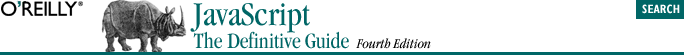home | O'Reilly's CD bookshelfs | FreeBSD | Linux | Cisco | Cisco Exam## 7.2. Functions as Data

This may not seem like a particularly interesting point to you unless you are familiar with languages like Java, in which functions are part of a program but cannot be manipulated by the program.

To understand how functions can be JavaScript data as well as JavaScript syntax, consider this function definition:

`function square(x) { return x*x; } `

This definition creates a new function object and assigns it to the variable square. The name of a function is really immaterial -- it is simply the name of a variable that holds the function. The function can be assigned to another variable and still work the same way:

```var a = square(4);  // a contains the number 16
var b = square;     // Now b refers to the same function that square does
var c = b(5);       // c contains the number 25 ```
```var o = new Object;
o.square = new Function("x", "return x*x");  // Note Function( ) constructor
y = o.square(16);                            // y equals 256 ```
```var a = new Array(3);
a = function(x) { return x*x; }  // Note function literal
a = 20;
a = a(a);                  // a contains 400 ```

The function invocation syntax in this last example looks strange, but it is still a legal use of the JavaScript ( ) operator!

Example 7-2 is a detailed example of the things that can be done when functions are used as data. It demonstrates how functions can be passed as arguments to other functions and also how they can be stored in associative arrays (which were introduced in Chapter 3 and are explained in detail in Chapter 8.) This example may be a little tricky, but the comments explain what is going on; it is worth studying carefully.

#### Example 7-2. Using functions as data

```// We define some simple functions here
function add(x,y) { return x + y; }
function subtract(x,y) { return x - y; }
function multiply(x,y) { return x * y; }
function divide(x,y) { return x / y; }

// Here's a function that takes one of the above functions
// as an argument and invokes it on two operands
function operate(operator, operand1, operand2)
{
return operator(operand1, operand2);
}

// We could invoke this function like this to compute the value (2+3) + (4*5):

// For the sake of example, we implement the functions again, this time
// using function literals. We store the functions in an associative array.
var operators = new Object( );
operators["add"] = function(x,y) { return x+y; };
operators["subtract"] = function(x,y) { return x-y; };
operators["multiply"] = function(x,y) { return x*y; };
operators["divide"] = function(x,y) { return x/y; };
operators["pow"] = Math.pow;  // Works for predefined functions too

// This function takes the name of an operator, looks up that operator
// in the array, and then invokes it on the supplied operands. Note
// the syntax used to invoke the operator function.
function operate2(op_name, operand1, operand2)
{
if (operators[op_name] == null) return "unknown operator";
else return operators[op_name](operand1, operand2);
}

// We could invoke this function as follows to compute
// the value ("hello" + " " + "world"):